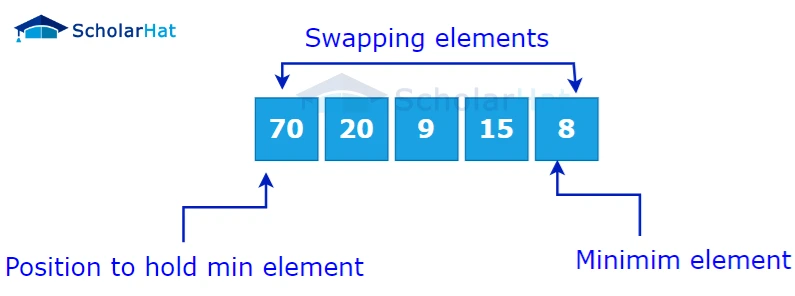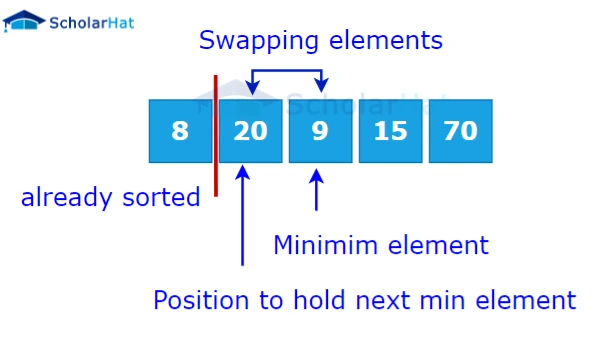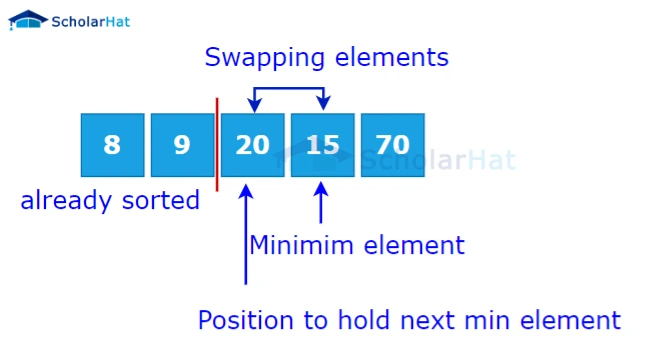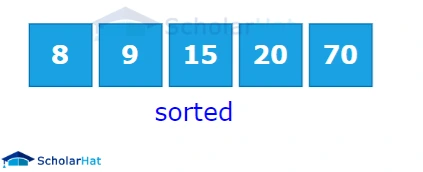# Selection Sort in Data Structures

Amit Kumar Ghosh  16 min read
12 Jun 2023
Intermediate
304 Views

## Introduction

Are you looking to be more knowledgeable about the intricacies of data structures? Selection sort is a sorting algorithm used in computer science that is relatively easy to understand, though figuring out its implementations can become complex. As someone who has studied selection sort and implemented it numerous times, I'm here to break down how selection sort works, its use cases, and how it compares to other common sorting algorithms. Read on for an introduction to understanding this powerful data structure!

## What is selection sort in data structure?

Selection sort in data structureis one of the fundamental sorting algorithms used in computer science. It is a simple, intuitive process that works by repeatedly selecting the smallest element from an unsorted list and moving it to the front of the list. This process is then repeated for the remaining unsorted elements until the list is fully sorted. Although not as efficient as other sorting algorithms such as quicksort or mergesort, selection sort still has its uses in certain situations. Understanding how these algorithms work is an essential part of mastering data structures, and selection sort is a great starting point for anyone interested in delving deeper into the world of computer science.

## How does selection sort work?

Selection sort is a simple sorting algorithm that works by repeatedly finding the minimum element from an unsorted list and moving it to the beginning (or end) of the list. Here are the steps:

1. Find the smallest element in the unsorted portion of the list.1. Swap it with the element at the beginning or end of the unsorted portion.1. Move the boundary of the unsorted portion of one element to the right or left.1. Repeat steps 1-3 until the entire list is sorted.#### Here is an example of selection sort in action: Unsorted list: 5, 2, 9, 3, 7

1. Find the smallest element in the unsorted portion (2).
2. Swap it with the first element to get: 2, 5, 9, 3, 7
3. Move the boundary of the unsorted portion to the right by one element.
4. Find the smallest element in the unsorted portion (3).
5. Swap it with the second element to get: 2, 3, 9, 5, 7
6. Move the boundary of the unsorted portion to the right by one element.
7. Find the smallest element in the unsorted portion (5).
8. Swap it with the third element to get: 2, 3, 5, 9, 7
9. Move the boundary of the unsorted portion to the right by one element.
10. Find the smallest element in the unsorted portion (7).
11. Swap it with the fourth element to get: 2, 3, 5, 7, 9
12. Move the boundary of the unsorted portion to the right by one element.
13. The list is now sorted.

Selection sort complexity

<>The time complexity of the selection sort is O(n^2), where "n" is the number of elements in the array. Selection sort works by repeatedly finding the minimum element from the unsorted part of the array and swapping it with the first element of the unsorted part. This Selection sort complexity process continues until the entire array is sorted. In the worst-case scenario, the array is sorted in reverse order, and for each element, we need to compare it with all the remaining elements in the unsorted part of the array. This means that we perform n-1 comparisons for the first element, n-2 comparisons for the second element, and so on, up to 1 comparison for the second-to-last element. The total number of comparisons is:
``` ```(n-1) + (n-2) + ... + 1
= n*(n-1)/2
= O(n^2)``````

Therefore, the time complexity of the selection sort is O(n^2), both in the average case and worst case. The space complexity of the selection sort is O(1), which means that it sorts the array in place.

Application of selection sort

### The applications of selection sort are:

1. Small Data Sets: Selection sort is best suited for small data sets, where the number of elements is relatively small. For such data sets, the time complexity of the selection sort is not a big issue, and it can provide a simple and efficient solution.
2. Education: Selection sort is a popular algorithm used in computer science education. It is often used as an example of a simple sorting algorithm that can be easily understood and implemented by beginners.
3. Embedded Systems: Selection sort is also used in embedded systems, where memory and processing power are limited. It is a simple algorithm that can be implemented in a small amount of code, making it a good choice for embedded systems.
4. Testing Other Sorting Algorithms: Selection sort is often used to test other sorting algorithms. Since selection sort is one of the simplest sorting algorithms, it is easy to implement and can be used as a benchmark to compare the performance of other, more complex sorting algorithms.
5. Partially Sorted Data: Selection sort performs well on partially sorted data. If a large portion of the input data is already sorted, selection sort will not perform unnecessary swaps, resulting in faster sorting times

## Selection sort algorithm

``` ```selectionSort(array, size)
repeat (size - 1) times
set the first unsorted element as the minimum
for each of the unsorted elements
if element < currentMinimum
set element as new minimum
swap minimum with first unsorted position
end selectionSort``````

``````
# Selection sort in Python

def selectionSort(array, size):

for step in range(size):
min_idx = step

for i in range(step + 1, size):

# to sort in descending order, change > to < in this line
# select the minimum element in each loop
if array[i] < array[min_idx]:
min_idx = i

# put min at the correct position
(array[step], array[min_idx]) = (array[min_idx], array[step])

data = [ 280, 117, 9, 12, 4]
size = len(data)
selectionSort(data, size)
print('Sorted Array in Ascending Order:')
print(data)

``````
``````
// Selection sort in Java

import java.util.Arrays;

class SelectionSort {
void selectionSort(int array[]) {
int size = array.length;

for (int step = 0; step < size - 1; step++) {
int min_idx = step;

for (int i = step + 1; i < size; i++) {

// To sort in descending order, change > to < in this line.
// Select the minimum element in each loop.
if (array[i] < array[min_idx]) {
min_idx = i;
}
}

// put min at the correct position
int temp = array[step];
array[step] = array[min_idx];
array[min_idx] = temp;
}
}

// driver code
public static void main(String args[]) {
int[] data = { 280, 117, 9, 12, 4 };
SelectionSort ss = new SelectionSort();
ss.selectionSort(data);
System.out.println("Sorted Array in Ascending Order: ");
System.out.println(Arrays.toString(data));
}
}``````
``````
// Selection sort in C++

#include <iostream>
using namespace std;

// function to swap the the position of two elements
void swap(int *a, int *b) {
int temp = *a;
*a = *b;
*b = temp;
}

// function to print an array
void printArray(int array[], int size) {
for (int i = 0; i < size; i++) {
cout << array[i] << " ";
}
cout << endl;
}

void selectionSort(int array[], int size) {
for (int step = 0; step < size - 1; step++) {
int min_idx = step;
for (int i = step + 1; i < size; i++) {

// To sort in descending order, change > to < in this line.
// Select the minimum element in each loop.
if (array[i] < array[min_idx])
min_idx = i;
}

// put min at the correct position
swap(&array[min_idx], &array[step]);
}
}

// driver code
int main() {
int data[] = {280, 117, 9, 12, 4};
int size = sizeof(data) / sizeof(data);
selectionSort(data, size);
cout << "Sorted array in Acsending Order:\n";
printArray(data, size);
}``````

Output

``````Sorted array in Acsending Order:
4, 9, 12, 117, 280``````

There are some advantages of selection sort, those are

• Simplicity: The selection sort is easy to understand and implement, making it a good choice for beginners or situations where simplicity is preferred over efficiency.
• Space efficiency: Selection sort requires minimal additional space beyond the input array, as it performs sorting in-place, which can be beneficial in situations where memory is limited.
• Stability: Selection sort is a stable sorting algorithm, meaning that it maintains the relative order of equal elements in the sorted output. This can be an important consideration when sorting data that contains duplicates.
• Performance on small datasets: Selection sort has a relatively low overhead compared to more advanced sorting algorithms, making it suitable for small datasets with a few hundred or thousand elements.
• Adaptive: Selection sort is adaptive, meaning that it performs better on partially sorted arrays than on completely unsorted arrays. This property can be useful in scenarios where data is added to an already sorted list or when only a few elements are out of order

There are some disadvantages of selection sort, those are

• Time complexity: The selection sort has a time complexity of O(n^2), where n is the number of elements in the array. This means that the algorithm becomes very slow as the size of the input array grows. For large arrays, selection sort can take a long time to complete.
• Not stable: Selection sort is not a stable sorting algorithm. A stable sorting algorithm is one that maintains the relative order of equal elements in the array. This means that if two elements are equal, their order in the sorted array should be the same as their order in the original array. However, selection sort does not guarantee this.
• Not adaptive: Selection sort is not an adaptive sorting algorithm. An adaptive sorting algorithm is one that can take advantage of the existing order in the array to improve its performance. However, selection sort does not consider the existing order of the array, and always performs the same number of comparisons and swaps.
• Inefficient for large datasets: Selection sort is inefficient for large datasets. When the array contains a large number of elements, selection sort can take a long time to complete. This makes it unsuitable for applications that require sorting large datasets.
• Not optimal: Selection sort is not an optimal sorting algorithm. An optimal sorting algorithm is one that can sort any input array in the minimum possible number of comparisons and swaps. However, selection sort does not always achieve the minimum number of comparisons and swaps required to sort an array.

Summary

While selection sort is not the most efficient for larger datasets, it remains an important tool in sorting data structures. Its simplicity makes it easy to understand and the fact that it requires only one pass through the data means that sorting can be finished quickly. With a few simple steps, you can easily implement a selection sort algorithm on your data. Furthermore, by understanding the fundamentals of selection sort and the principles behind it, you’ll be able to apply the same concept to other sorting algorithms as well. This ensures that you can keep your data structures organized with no issues at all. So why wait? Try implementing selection sort today and see just how powerful this algorithm can be!

Similar Articles